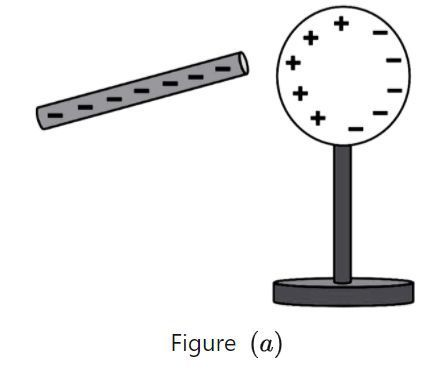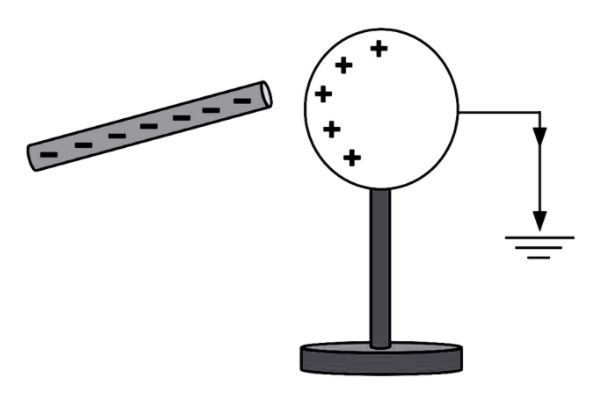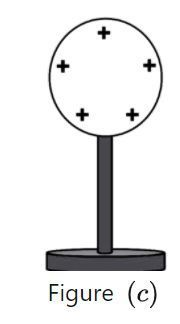Home/Class 12/Physics/

## QuestionPhysicsClass 12

How can you charge a metal sphere positively without touching it?

By using electrostatic induction
4.64.6## Solution

A metal sphere can be charged positively without touching by means of electrostatic induction.
$$1.$$ A negatively charged rod is brought near the metal sphere mounted on an insulating stand as shown in   . The rod is full of negative charges alone. Positive charges are induced on the surface of the sphere which is near the negatively charged rod. The negative charges of the sphere move away to the farther end due to repulsion. And the nearer end of the sphere becomes positively charged with the shortfall of electrons.
shown in $$\mathit{Fig.}(a)$$$$2.$$ Then connect the sphere to the ground using a conducting wire as shown in  $$\mathit{Fig.}(b).$$  The free electrons will start to flow into the ground from the sphere.Figure $$(b)$$
$$3\ldotp$$Now disconnect the sphere from the ground and remove the rod and we can
observe the uniform spread of positive charges over the sphere as shown in  $$\mathit{Fig.}(c)$$ .Thus charging a metal sphere positively is achieved without touching it by means of induction.